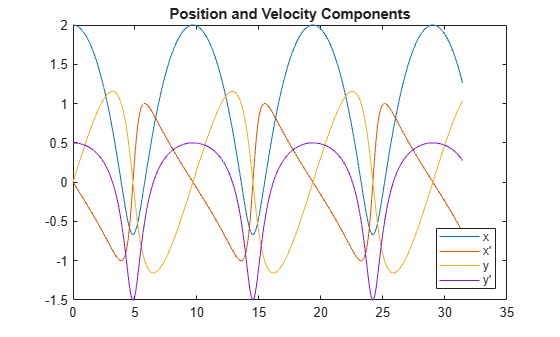# ode89

## 语法

[t,y] = ode89(odefun,tspan,y0)
[t,y] = ode89(odefun,tspan,y0,options)
[t,y,te,ye,ie] = ode89(odefun,tspan,y0,options)
sol = ode89(___)

## 说明

[t,y] = ode89(odefun,tspan,y0)（其中 tspan = [t0 tf]）求微分方程组 $y\text{'}=f\left(t,y\right)$ 从 t0 到 tf 的积分，初始条件为 y0。解数组 y 中的每一行都与列向量 t 中返回的值相对应。所有 MATLAB® ODE 求解器都可以解算 $y\text{'}=f\left(t,y\right)$ 形式的方程组，或涉及质量矩阵 $M\left(t,y\right)y\text{'}=f\left(t,y\right)$ 的问题。求解器使用类似的语法。ode23s 求解器只能解算质量矩阵为常量的问题。ode15s 和 ode23t 可以解算具有奇异质量矩阵的问题，称为微分代数方程 (DAE)。使用 odeset 的 Mass 选项指定质量矩阵。
[t,y] = ode89(odefun,tspan,y0,options) 还使用由 options（使用 odeset 函数创建的参数）定义的积分设置。例如，设置 AbsTol 和 RelTol 选项指定绝对误差容限和相对误差容限，或者设置 Mass 选项提供质量矩阵。
[t,y,te,ye,ie] = ode89(odefun,tspan,y0,options) 还求 (t,y) 的函数（称为事件函数）在何处为零。在输出中，te 是事件的时间，ye 是事件发生时的解，ie 是触发的事件的索引。对于每个事件函数，应指定积分是否在零点处终止以及过零方向是否重要。为此，请将 odeset 的 'Events' 选项设置为函数（例如 myEventFcn 或 @myEventFcn），并创建一个对应的函数：[value,isterminal,direction] = myEventFcn(,,t,y)。有关详细信息，请参阅 ODE 事件位置。
sol = ode89(___) 返回一个结构体，您可以将该结构体与 deval 结合使用来计算区间 [t0 tf] 中任意点位置的解。您可以使用上述语法中的任何输入参数组合。

## 示例

${y}^{\prime }=2t.$

tspan = [0 5]; y0 = 0; [t,y] = ode89(@(t,y) 2*t, tspan, y0);

plot(t,y,'-o')van der Pol 方程为二阶 ODE

${y}_{1}^{\prime \prime }-\mu \left(1-{y}_{1}^{2}\right){y}_{1}^{\prime }+{y}_{1}=0,$

$\begin{array}{cl}{y}_{1}^{\prime }& ={y}_{2}\\ {y}_{2}^{\prime }& =\mu \left(1-{y}_{1}^{2}\right){y}_{2}-{y}_{1}.\end{array}$

function dydt = vdp1(t,y) %VDP1 Evaluate the van der Pol ODEs for mu = 1 % % See also ODE113, ODE23, ODE45. % Jacek Kierzenka and Lawrence F. Shampine % Copyright 1984-2014 The MathWorks, Inc. dydt = [y(2); (1-y(1)^2)*y(2)-y(1)]; 

[t,y] = ode89(@vdp1,[0 20],[2; 0]);

plot(t,y(:,1),'-o',t,y(:,2),'-o') title('Solution of van der Pol Equation (\mu = 1) with ODE89'); xlabel('Time t'); ylabel('Solution y'); legend('y_1','y_2')ode89 仅适用于使用两个输入参数（ty）的函数。但是，通过在函数外部定义参数并在指定函数句柄时传递这些参数，可以传入额外参数。

${y}^{\prime \prime }=\frac{A}{B}ty.$

$\begin{array}{cl}{y}_{1}^{\prime }& ={y}_{2}\\ {y}_{2}^{\prime }& =\frac{A}{B}t{y}_{1}.\end{array}$

function dydt = odefcn(t,y,A,B) dydt = zeros(2,1); dydt(1) = y(2); dydt(2) = (A/B)*t.*y(1); end 

A = 1; B = 2; tspan = [0 5]; y0 = [0 0.01]; [t,y] = ode89(@(t,y) odefcn(t,y,A,B), tspan, y0);

plot(t,y(:,1),'-o',t,y(:,2),'-.')function dydt = odefcn(t,y,A,B) dydt = zeros(2,1); dydt(1) = y(2); dydt(2) = (A/B)*t.*y(1); end

ode45 相比，ode113ode78ode89 求解器可以更好地求解具有严格误差容限的问题。这些求解器更胜一筹的常见情况是在轨道动力学问题中，这些问题的解曲线是平滑的且在求解器的每个时间步中都需要很高的准确度。

$\begin{array}{l}{{\mathit{x}}^{\prime }}^{\prime }=-\mathit{x}/{\mathit{r}}^{3}\\ {{\mathit{y}}^{\prime }}^{\prime }=-\mathit{y}/{\mathit{r}}^{3},\end{array}$

$\mathit{r}=\sqrt{{\mathit{x}}^{2}+{\mathit{y}}^{2}}.$

$\begin{array}{l}{\mathit{y}}_{1}=\mathit{x}\\ {\mathit{y}}_{2}={\mathit{x}}^{\prime }\\ {\mathit{y}}_{3}=\mathit{y}\\ {\mathit{y}}_{4}={\mathit{y}}^{\prime }.\end{array}$

$\begin{array}{l}{{\mathit{y}}_{1}}^{\prime }={\mathit{y}}_{2}\\ {{\mathit{y}}_{2}}^{\prime }=-{\mathit{y}}_{1}/{\mathit{r}}^{3}\\ {{\mathit{y}}_{3}}^{\prime }={\mathit{y}}_{4}\\ {{\mathit{y}}_{4}}^{\prime }=-{\mathit{y}}_{3}/{\mathit{r}}^{3}.\end{array}$

function dy = twobodyode(t,y) % Two-body problem with one mass much larger than the other. r = sqrt(y(1)^2 + y(3)^2); dy = [y(2); -y(1)/r^3; y(4); -y(3)/r^3]; end 

opts = odeset('Reltol',1e-13,'AbsTol',1e-14,'Stats','on'); tspan = [0 10*pi]; y0 = [2 0 0 0.5]; [t,y] = ode89(@twobodyode, tspan, y0, opts);
243 successful steps 0 failed attempts 5103 function evaluations 
plot(t,y) legend('x','x''','y','y''','Location','SouthEast') title('Position and Velocity Components')figure plot(y(:,1),y(:,3),'-o',0,0,'ro') axis equal title('Orbit of Smaller Mass')ode45 相比，ode89 求解器能够通过更少的步骤和函数计算更快地获得解。

function dy = twobodyode(t,y) % Two-body problem with one mass much larger than the other. r = sqrt(y(1)^2 + y(3)^2); dy = [y(2); -y(1)/r^3; y(4); -y(3)/r^3]; end

## 输入参数

function dydt = odefun(t,y) dydt = 5*y-3; end

$\begin{array}{l}y{\text{'}}_{1}={y}_{1}+2{y}_{2}\\ y{\text{'}}_{2}=3{y}_{1}+2{y}_{2}\end{array}$

function dydt = odefun(t,y) dydt = zeros(2,1); dydt(1) = y(1)+2*y(2); dydt(2) = 3*y(1)+2*y(2); end

• 如果 tspan 有两个元素 [t0 tf]，求解器将返回在该区间内的每个内部积分步计算的解。

• 如果 tspan 包含两个以上的元素，[t0,t1,t2,...,tf]，求解器将返回在给定点处计算的解。但是，求解器不会精确步进到 tspan 中指定的每个点。此时，求解器使用自己的内部积分步来计算解，然后在 tspan 中请求的各点处计算解。在指定点处生成的解与在每个内部积分步计算的解具有相同的准确度级别。

指定多个中间点对计算效率影响甚微，但对于大型系统可能会影响内存管理。

• 如果 tspan 包含多个中间点 [t0,t1,t2,...,tf]，则指定的点表示了问题的规模，这可能影响求解器使用的 InitialStep 的值。因此，根据您是将 tspan 指定为二元素向量还是包含中间点的向量，求解器获得的解可能有所不同。

• tspan 中的初始值和最终值用于计算最大步长 MaxStep。因此，更改 tspan 中的初始值或最终值可能导致求解器使用不同步长序列，从而可能会更改解。

options 结构体，指定为结构体数组。使用 odeset 函数创建或修改 options 结构体。有关与每个求解器兼容的选项列表，请参阅 ODE 选项摘要

## 输出参数

• 如果 tspan 包含两个元素 [t0 tf]，则 t 包含用于执行积分的内部计算点。

• 如果 tspan 包含两个以上元素，则 ttspan 相同。

sol.x

sol.y

sol.solver

sol.xe

sol.ye

sol.xe 中的事件相对应的解。

sol.ie

Events 选项中指定的函数所返回的向量的索引。这些值指示求解器检测到的事件。

## 算法

ode89 是 Verner 的“最稳健”的 8 阶连续外推 Runge-Kutta 9(8) 法的一种实现。解的精度提升到了 9 阶。8 阶连续外推需要对 odefun 进行五次额外计算，但仅限于需要插值的步。

 Verner, J. H. “Numerically Optimal Runge–Kutta Pairs with Interpolants.” Numerical Algorithms 53, no. 2–3 (March 2010): 383–396. https://doi.org/10.1007/s11075-009-9290-3.